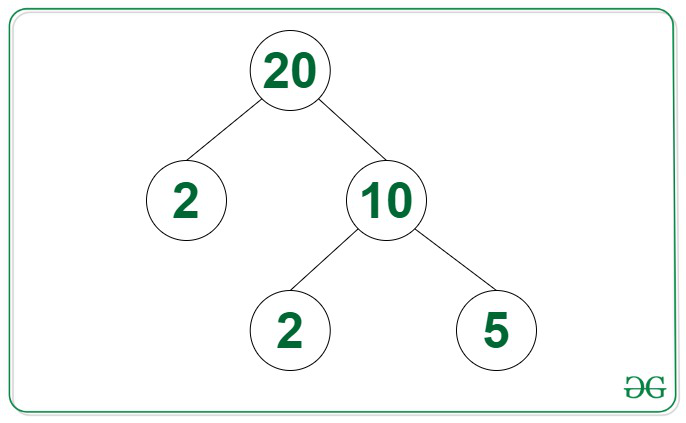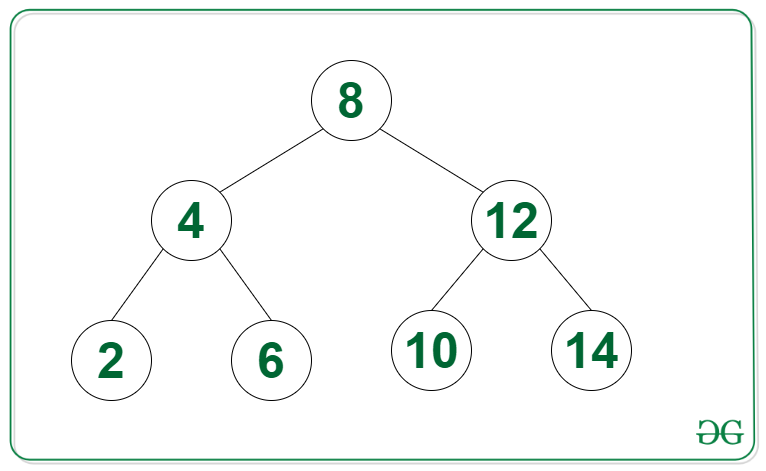GeeksforGeeks App
Open AppBrowser
Continue

# Count of nodes in a binary tree having their nodes in range [L, R]

Given a Binary Tree consisting of N nodes and two positive integers L and R, the task is to find the count of nodes having their value in the range [L, R].

Examples:

Input: Tree in the image below, L = 4, R = 15Output: 2
Explanation: The nodes in the given Tree that lies in the range [4, 15] are {5, 10}.

Input: Tree in the image below, L = 8, R = 20Output: 4

Approach: The given problem can be solved by performing any Tree Traversal and maintaining the count of nodes having their values in the range [L, R]. This article uses a DFS traversal.

Below is the implementation of the above approach:

## C++

 `// C++ program for the above approach``#include ``using` `namespace` `std;` `// Class for node of the Tree``class` `Node {``public``:``    ``int` `val;``    ``Node *left, *right;``};` `// Function to create a new Binary node``Node* newNode(``int` `item)``{``    ``Node* temp = ``new` `Node();``    ``temp->val = item;``    ``temp->left = temp->right = NULL;` `    ``// Return the newly created node``    ``return` `temp;``}` `// Function to find the count of``// nodes in the given tree with``// their value in the range [1, N]``int` `countRange(Node* root, ``int` `low,``               ``int` `high, ``int` `count)``{``    ``int` `val = 0;` `    ``// If root exists``    ``if` `(root != NULL) {` `        ``val += root->val >= low``                       ``&& root->val <= high``                   ``? 1``                   ``: 0;``    ``}` `    ``// Otherwise return``    ``else` `{``        ``return` `0;``    ``}` `    ``// Add count of current node,``    ``// count in left subtree, and``    ``// count in the right subtree``    ``count = val``            ``+ countRange(root->left,``                         ``low, high, count)``            ``+ countRange(root->right,``                         ``low, high, count);` `    ``// Return Answer``    ``return` `count;``}` `// Driver Code``int` `main()``{``    ``Node* root = NULL;``    ``root = newNode(20);``    ``root->left = newNode(2);``    ``root->right = newNode(10);``    ``root->right->left = newNode(2);``    ``root->right->right = newNode(5);` `    ``int` `L = 4, R = 15;``    ``cout << countRange(root, L, R, 0);` `    ``return` `0;``}`

## Java

 `// Java implementation Count of nodes in``// a binary tree having their nodes in range [L, R]``import` `java.util.*;` `public` `class` `GFG{``    ``// structure of tree node``    ``static` `class` `Node {``        ``int` `val;``        ``Node left, right;``    ``};` `    ``// return a new binary tree node``    ``static` `Node newNode(``int` `item){``        ``Node temp = ``new` `Node();``        ``temp.val = item;``        ``temp.left = temp.right = ``null``;``        ``return` `temp;``    ``}` `    ``// Function to find the count of``    ``// nodes in the given tree with``    ``// their value in the range [1, N]``    ``static` `int` `countRange(Node root, ``int` `low, ``int` `high, ``int` `count){``        ``int` `val = ``0``;``        ``// If root exists``        ``if` `(root != ``null``) {``            ``val += root.val >= low && root.val <= high ? ``1` `: ``0``;``        ``}``else` `{``          ``return` `0``;``        ``}` `        ``// Add count of current node,``        ``// count in left subtree, and``        ``// count in the right subtree``        ``count = val + countRange(root.left, low, high, count)``                      ``+ countRange(root.right, low, high, count);` `        ``return` `count;``    ``}` `  ``// Driver Code``  ``public` `static` `void` `main(String[] args){``    ``Node root = ``null``;``    ``root = newNode(``20``);``    ``root.left = newNode(``2``);``    ``root.right = newNode(``10``);``    ``root.right.left = newNode(``2``);``    ``root.right.right = newNode(``5``);` `    ``int` `L = ``4``, R = ``15``;``    ``System.out.print(countRange(root, L, R, ``0``));``  ``}``}` `// This code is contributed by Yash Agarwal`

## Python3

 `# Python program for the above approach` `# Class for Node of the Tree``class` `Node:``    ``def` `__init__(``self``, val):``        ``self``.val ``=` `val;``        ``self``.left ``=` `None``;``        ``self``.right ``=` `None``;` `# Function to create a new Binary Node``def` `newNode(item):``    ``temp ``=` `Node(item);``    ` `    ``# Return the newly created Node``    ``return` `temp;` `# Function to find the count of``# Nodes in the given tree with``# their value in the range [1, N]``def` `countRange(root, low, high, count):``    ``val ``=` `0``;` `    ``# If root exists``    ``if` `(root !``=` `None``):` `        ``val ``+``=` `1` `if``(root.val >``=` `low ``and` `root.val <``=` `high) ``else` `0``;`  `    ``# Otherwise return``    ``else``:``        ``return` `0``;` `    ``# Add count of current Node,``    ``# count in left subtree, and``    ``# count in the right subtree``    ``count ``=` `val ``+` `countRange(root.left, low, high, count) ``+` `countRange(root.right, low, high, count);` `    ``# Return Answer``    ``return` `count;`  `# Driver Code``if` `__name__ ``=``=` `'__main__'``:``    ``root ``=` `None``;``    ``root ``=` `newNode(``20``);``    ``root.left ``=` `newNode(``2``);``    ``root.right ``=` `newNode(``10``);``    ``root.right.left ``=` `newNode(``2``);``    ``root.right.right ``=` `newNode(``5``);` `    ``L ``=` `4``;``    ``R ``=` `15``;``    ``print``(countRange(root, L, R, ``0``));` `# This code is contributed by 29AjayKumar`

## C#

 `// C# program for the above approach``using` `System;` `public` `class` `GFG{` `  ``// Class for node of the Tree``  ``class` `Node {` `    ``public` `int` `val;``    ``public` `Node left, right;``  ``};` `  ``// Function to create a new Binary node``  ``static` `Node newNode(``int` `item)``  ``{``    ``Node temp = ``new` `Node();``    ``temp.val = item;``    ``temp.left = temp.right = ``null``;` `    ``// Return the newly created node``    ``return` `temp;``  ``}` `  ``// Function to find the count of``  ``// nodes in the given tree with``  ``// their value in the range [1, N]``  ``static` `int` `countRange(Node root, ``int` `low,``                        ``int` `high, ``int` `count)``  ``{``    ``int` `val = 0;` `    ``// If root exists``    ``if` `(root != ``null``) {` `      ``val += root.val >= low``        ``&& root.val <= high``        ``? 1``        ``: 0;``    ``}` `    ``// Otherwise return``    ``else` `{``      ``return` `0;``    ``}` `    ``// Add count of current node,``    ``// count in left subtree, and``    ``// count in the right subtree``    ``count = val``      ``+ countRange(root.left,``                   ``low, high, count)``      ``+ countRange(root.right,``                   ``low, high, count);` `    ``// Return Answer``    ``return` `count;``  ``}` `  ``// Driver Code``  ``public` `static` `void` `Main(String[] args)``  ``{``    ``Node root = ``null``;``    ``root = newNode(20);``    ``root.left = newNode(2);``    ``root.right = newNode(10);``    ``root.right.left = newNode(2);``    ``root.right.right = newNode(5);` `    ``int` `L = 4, R = 15;``    ``Console.Write(countRange(root, L, R, 0));``  ``}``}` `// This code is contributed by shikhasingrajput`

## Javascript

 ``

Output

`2`

Time Complexity : O(n), where n is the number of nodes in the tree
Auxiliary Space: O(h), where h is the height of the tree.

Another Approach(Iterative):
The given problem can be solved by using the Level Order Traversal

. Follow the steps below to solve the problem:

1) Create a queue(q) and initialize count variable with 0, and store the nodes in q along wise level order and iterate for next level.
2) Perform level order traversal and check if current node value lie in range then increment the count variable.
3) After completing the above steps, return count variable.

Below is the implementation of above approach:

## C++

 `// C++ program for the above approach``#include``using` `namespace` `std;` `// struct of binary tree node``struct` `Node{``    ``int` `data;``    ``Node* left;``    ``Node* right;``};` `// function to create a new node``Node* newNode(``int` `data){``    ``Node* temp = ``new` `Node();``    ``temp->data = data;``    ``temp->left = temp->right = NULL;``    ``return` `temp;``}` `// Function to find the count of``// nodes in the given tree with``// their value in the range [1, N]``int` `countRange(Node* root, ``int` `low, ``int` `high){``    ``int` `count = 0;``    ` `    ``// if root exists``    ``if``(root == NULL) ``return` `count;``    ``queue q;``    ``q.push(root);``    ``while``(!q.empty()){``        ``Node* temp = q.front();``        ``q.pop();``        ``if``(temp->data <= high && temp->data >= low){``            ``count++;``        ``}``        ``if``(temp->left != NULL) q.push(temp->left);``        ``if``(temp->right != NULL) q.push(temp->right);``    ``}``    ``return` `count;``}` `//driver code``int` `main(){``    ``Node *root = newNode(20);``    ``root->left = newNode(2);``    ``root->right = newNode(10);``    ``root->right->left = newNode(2);``    ``root->right->right = newNode(5);`` ` `    ``int` `L = 4, R = 15;``    ``cout << countRange(root, L, R);`` ` `    ``return` `0;``}` `// This code is contributed by Kirti Agarwal(kirtiagarwal23121999)`

## Java

 `import` `java.util.LinkedList;``import` `java.util.Queue;` `class` `Node {``  ``int` `data;``  ``Node left, right;``  ``Node(``int` `data)``  ``{``    ``this``.data = data;``    ``left = right = ``null``;``  ``}``}` `public` `class` `Main {``  ``// Function to find the count of``  ``// nodes in the given tree with``  ``// their value in the range [low, high]``  ``public` `static` `int` `countRange(Node root, ``int` `low,``                               ``int` `high)``  ``{``    ``int` `count = ``0``;``    ``// if root exists``    ``if` `(root == ``null``)``      ``return` `count;``    ``Queue q = ``new` `LinkedList<>();``    ``q.offer(root);``    ``while` `(!q.isEmpty()) {``      ``Node temp = q.poll();``      ``if` `(temp.data <= high && temp.data >= low) {``        ``count++;``      ``}``      ``if` `(temp.left != ``null``)``        ``q.offer(temp.left);``      ``if` `(temp.right != ``null``)``        ``q.offer(temp.right);``    ``}``    ``return` `count;``  ``}` `  ``// driver code``  ``public` `static` `void` `main(String[] args)``  ``{``    ``Node root = ``new` `Node(``20``);``    ``root.left = ``new` `Node(``2``);``    ``root.right = ``new` `Node(``10``);``    ``root.right.left = ``new` `Node(``2``);``    ``root.right.right = ``new` `Node(``5``);` `    ``int` `L = ``4``, R = ``15``;``    ``System.out.println(countRange(root, L, R));``  ``}``}` `// This code is contributed by aadityamaharshi21.`

## Python3

 `# Python program for the above approach``class` `Node:``    ``def` `__init__(``self``, data):``        ``self``.data ``=` `data``        ``self``.left ``=` `None``        ``self``.right ``=` `None``        ` `def` `newNode(data):``    ``temp ``=` `Node(data)``    ``return` `temp` `def` `countRange(root, low, high):``    ``count ``=` `0``    ``if` `root ``is` `None``:``        ``return` `count``    ``q ``=` `[]``    ``q.append(root)``    ``while` `len``(q) > ``0``:``        ``temp ``=` `q.pop(``0``)``        ``if` `temp.data <``=` `high ``and` `temp.data >``=` `low:``            ``count ``+``=` `1``        ``if` `temp.left ``is` `not` `None``:``            ``q.append(temp.left)``        ``if` `temp.right ``is` `not` `None``:``            ``q.append(temp.right)``    ``return` `count` `root ``=` `newNode(``20``)``root.left ``=` `newNode(``2``)``root.right ``=` `newNode(``10``)``root.right.left ``=` `newNode(``2``)``root.right.right ``=` `newNode(``5``)` `L ``=` `4``R ``=` `15``print``(countRange(root, L, R))` `# This code is contributed by shivamsharma215`

## C#

 `using` `System;``using` `System.Collections.Generic;` `class` `Program``{``    ``// Definition for a binary tree node``    ``class` `Node``    ``{``        ``public` `int` `data;``        ``public` `Node left;``        ``public` `Node right;``    ``}` `    ``// Function to create a new node``    ``static` `Node newNode(``int` `data)``    ``{``        ``Node temp = ``new` `Node();``        ``temp.data = data;``        ``temp.left = temp.right = ``null``;``        ``return` `temp;``    ``}` `    ``// Function to count the number of nodes in the given range``    ``static` `int` `countRange(Node root, ``int` `low, ``int` `high)``    ``{``        ``int` `count = 0;``        ``// If the root is null, return 0``        ``if` `(root == ``null``) ``return` `count;` `        ``Queue q = ``new` `Queue();``        ``q.Enqueue(root);``        ``// Use BFS to traverse the tree``        ``while` `(q.Count != 0)``        ``{``            ``Node temp = q.Dequeue();``            ``// Check if the data of the node is in the given range``            ``if` `(temp.data <= high && temp.data >= low)``            ``{``                ``count++;``            ``}``            ``// Enqueue the left and right children if they exist``            ``if` `(temp.left != ``null``) q.Enqueue(temp.left);``            ``if` `(temp.right != ``null``) q.Enqueue(temp.right);``        ``}``        ``return` `count;``    ``}` `    ``static` `void` `Main(``string``[] args)``    ``{``        ``// Create the binary tree``        ``Node root = newNode(20);``        ``root.left = newNode(2);``        ``root.right = newNode(10);``        ``root.right.left = newNode(2);``        ``root.right.right = newNode(5);` `        ``int` `L = 4, R = 15;``        ``Console.WriteLine(countRange(root, L, R));``    ``}``}`

## Javascript

 `//create a new node` `class Node {``    ``constructor(data) {``        ``this``.data = data;``        ``this``.left = ``null``;``        ``this``.right = ``null``;``    ``}``}``// Function to find the count of``// nodes in the given tree with``// their value in the range [1, N]``function` `countRange(root, low, high) {``    ``let count = 0;``    ``if` `(!root) ``return` `count;` `    ``let queue = [];``    ``queue.push(root);` `    ``while` `(queue.length > 0) {``        ``let temp = queue.shift();``        ``if` `(temp.data <= high && temp.data >= low) {``            ``count++;``        ``}``        ``if` `(temp.left) queue.push(temp.left);``        ``if` `(temp.right) queue.push(temp.right);``    ``}` `    ``return` `count;``}` `let root = ``new` `Node(20);``root.left = ``new` `Node(2);``root.right = ``new` `Node(10);``root.right.left = ``new` `Node(2);``root.right.right = ``new` `Node(5);` `let low = 4;``let high = 15;``console.log(countRange(root, low, high));`

Output

`2`

Time Complexity: O(N) where N is the number of nodes in given binary tree.
Auxiliary Space: O(N) due to queue data structure.

My Personal Notes arrow_drop_up